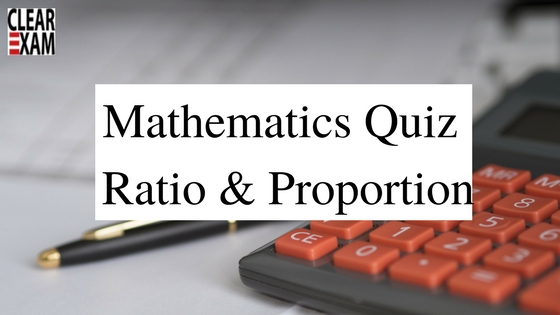## CTET and KVS Exam Quiz on Mathematics (Ratio & Proportion)

Mathematics is an important subject in CTET & KVS Exam. In any of teaching exam, Mathematics carries weightage of 22-25 %  of questions, with focused practice good marks can be fetched from this section. These questions are very important in achieving your success in CTET, KVS and Other TET Exams.

1.The sum of three numbers is 58. The ratio of first to second is 4: 9 and the second to third is 9: 16, the sum of first and third numbers is  -
(a)40 (b)29
(c)28 (d)50

2. Two numbers are in the ratio 3: 5. If 9 is subtracted from each, the new numbers are in the ratio of 12: 13. Then, smaller number is -
(a)27 (b)33
(c)49 (d)55

3.The mean proportional between the numbers 8 and 18 is
(a)32/9 (b)12
(c)13 (d)None of these

4.Find the third proportional to 9 and 12.
(a)12 (b)14
(c)16 (d)18

5.Find the fourth proportional to 4, 5 and 12.
(a)13 (b)14
(c)15 (d)16

6.A bag contains Rs. 102 in the form of rupee, 50 paise and 10 paise coins in the ratio 3: 4: 10. The number of 10 paise coins is -
(a)340 (b)60
(c)80 (d)170

7.A sum of Rs. 53 is divided amongst A, B and C in such a way that A gets Rs. 7 more than B gets and B gets Rs. 8 more than C gets, the ratio of their shares is-
(a)6 : 7 : 8 (b)25 : 18 : 10
(c)12: 14: 9 (d)None of these

8.In a school, the number of boys and that of the girls are in the respective ratio of 2 : 3. If the number of boys is increased by 20% and that of girls is increased by 10%, what will be the new ratio of numbers of boys to that of the girls?
(a)14: 5 (b) 5: 8
(c)13: 4 (d) 8: 11

9.If a sum of money is to be divided among A, B and C such that A’s share is equal to twice of B’s share and B’s share is 4 times of C’s share, then their shares are in the ratio -
(a)1 : 2 : 4 (b)1 : 4 : 1
(c)8 : 4 : 1 (d) 2: 4: 1

10.A and B start a business by investing Rs. 1,00,000 and Rs. 150000 respectively. Find the share of each out of a total profit of Rs. 24000.
(a)9000, 15000 (b)9600, 14400

(c)12000, 12000 (d)11600, 12400

1. (a) 2. (b) 3. (b) 4. (c) 5. (c) 6. (d) 7. (b) 8. (d) 9. (c) 10. (b)

Thanks
Team Clear CtetWant to Know More
Please fill in the details below:

Name

ltr
item
Clear CTET - Coaching Institute for CTET, DSSSB and KVS: CTET and KVS Exam Quiz on Mathematics (Ratio & Proportion)
CTET and KVS Exam Quiz on Mathematics (Ratio & Proportion)
Attempt here quiz on Mathematics (Ratio & Proportion) for CTET, KVS and Other TET Exams.
https://4.bp.blogspot.com/-lNqtOoSf8IM/Wi4wZWry5nI/AAAAAAAAAvw/-nlbrtKG2LEEP37b2yhTwrkcR-EDzTD1wCLcBGAs/s1600/Mathematics-Percentage-Quiz%2B%25281%2529.jpg
https://4.bp.blogspot.com/-lNqtOoSf8IM/Wi4wZWry5nI/AAAAAAAAAvw/-nlbrtKG2LEEP37b2yhTwrkcR-EDzTD1wCLcBGAs/s72-c/Mathematics-Percentage-Quiz%2B%25281%2529.jpg
Clear CTET - Coaching Institute for CTET, DSSSB and KVS
https://www.clearctet.com/2017/12/ctet-and-kvs-exam-quiz-on-mathematics_11.html
https://www.clearctet.com/
https://www.clearctet.com/
https://www.clearctet.com/2017/12/ctet-and-kvs-exam-quiz-on-mathematics_11.html
true
5140436504468951946
UTF-8